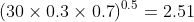Scan QR code or get instant email to install app

Question:

# The probability that it will rain on any given day in the month of June (30 days) is 0.3. The expected value and standard deviation of the number of rainy days in June are closest to:Expected Value and Standard Deviation

A 9 and 2.51
explanation

1212Using the binomial distribution:
Expected Value = 30 x 0.3 = 9
Standard Deviation =